esson: Divisibility Check
 Basics of Radicals When we simplify square roots, it is important to understand the fundamentals using the basics. What is this expression equal to?It is equal to 3, but why? Here is the reason.So, we can see squaring is an inverse operation with square roots. Similarly, look at this problem with a cube root.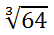The answer is 4. Here is the reason.It is evident that cubing and cube rooting are inverse operations.
 Parts of a Radical Radicals have several parts. Let us review the parts of a radical expression.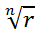The symbol that looks like a checkmark is called a radical. A number under the radical, marked with the letter 'r,' refers to the radicand. The small number written by the checkmark, marked with the letter 'n,' refers to the index.     If an index is not written, it is assumed to be a 2 and is a square root radical.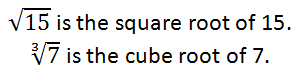Steps for Simplifying Radical Expressions To simplify radical expressions, here are the steps within this graphic organizer:Simplifying Radical Expressions: Numbers The best way to learn how to simplify an expression is to examine an example. Example 1: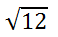This problem does not have a perfect answer. If we were to use a calculator to find the solution, it would provide us with a decimal solution somewhere between 3 and 4. Our only other option is to simplify the radical using the steps outlined within our graphic organizer in the last section, Steps for Simplifying Radical Expressions.     We will begin with a factor tree. We will break up the 12 as 3 times 4.If a prime number is found, circle it. 3 is prime because it has no other smaller factors other than 1. However, 4 is not prime (called composite) and it can be factored as follows.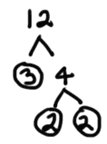So, we will write these in place of the 12 under the radical.Since the index is not written, it is 2. This means we group the factors in groups of two, like so.For each group, a factor comes out. Anything ungrouped will remain under the radical, like so.ideo: Simplifying Radicalsuiz: Simplifying Radical Expressions Example 2:This is a factor tree for the radicand 72.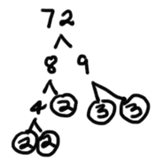Now, we will write the prime factors under a radical.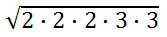The next step is to group the factors. The index is 2, so we will group in pairs.Place a factor on the outside of the radical for each group.Next, clean up the problem to get the final answer.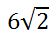ideo: Simplifying Radicalsuiz: Simplifying Radical Expressions     Go to the next section to see examples with cube roots. Simplifying Radical Expressions: Numbers and Variables Here are some examples that contain numbers and variables. Example 1:The factor tree is not shown, but here are the factors of 400.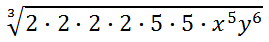Let us also write all the factors of the letters.We will now use the index 3 to remind us to group the factors by triples, like so.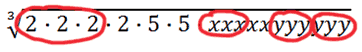For each group, place one factor outside of the radical. All ungrouped factors remain inside the radical.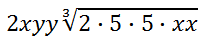Multiplying the factors to clean up the expression, we get...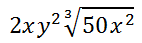Clearly, we can see that the variables add no more complexity to simplifying radical expressions. Example 2:First, write the prime factors of the radicand.Next, group the factors by quadruplets because the index is 4.Next, place a factor outside the radical for each group. Ungrouped factors remain inside the radical.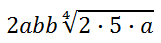Now, we need to clean up the problem for the final solution.Adding and Subtracting Radicalsideo: Adding and Subtracting Radicals Multiplying Radicalsideo: Multiplying Radicals Instructional Videos Try these videos, which can help you learn the content above.ideo: Simplifying Radicalsideo: Adding and Subtracting Radicalsideo: Multiplying Radicals Interactive Quizmaster Try this quiz, which can help you learn the content above.uiz: Simplifying Radical Expressions Related Lessons and Videos Try these lessons, which are related to the sections above.esson: Divisibility Checkesson: Complex Numbersesson: Solving Radical Equationsideo: Simplifying Radical Expressions: Complex Numbers